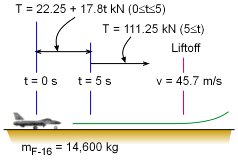Ch 4. Particle Momentum and Impulse Multimedia Engineering Dynamics Impulse & Momentum Consv. Linear Momentum Impact AngularMomentum MassFlow
 Chapter - Particle - 1. General Motion 2. Force & Accel. 3. Energy 4. Momentum - Rigid Body - 5. General Motion 6. Force & Accel. 7. Energy 8. Momentum 9. 3-D Motion 10. Vibrations Appendix Basic Math Units Basic Equations Sections Search eBooks Dynamics Fluids Math Mechanics Statics Thermodynamics Author(s): Kurt Gramoll ©Kurt GramollDYNAMICS - CASE STUDY IntroductionProblem Diagram The pilot of an F-16 must get his plane in the air in less than 30 seconds to defend against enemy aircraft. Can he do it? What is known: The mass of the F-16 is m = 14,600 kg. The liftoff speed is vLO = 45.7 m/s. For the first 5 seconds of the takeoff roll, the magnitude of the thrust of the jet increases from 22,250 N to 111,250 N at a constant rate. After 5 seconds, the thrust is constant at 111,250 N. The takeoff roll is a straight line. All friction forces can be neglected. Questions What is the velocity of the F-16 after 5 seconds? How long does it take for the F-16 to reach the velocity required for liftoff? Approach Express the thrust of the F-16 as a function of time. Determine the relationship between the external force acting on the plane and the change in velocity. Use the principle of impulse and momentum.

Practice Homework and Test problems now available in the 'Eng Dynamics' mobile app
Includes over 400 problems with complete detailed solutions.
Available now at the Google Play Store and Apple App Store.